# Quantum fermions from classical statistics quantum mechanics can

• Slides: 72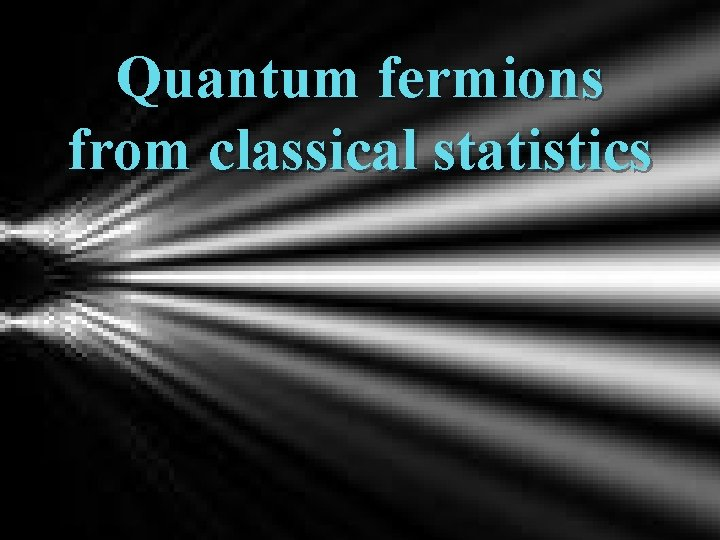Quantum fermions from classical statisticsquantum mechanics can be described by classical statistics !quantum particle from classical probabilities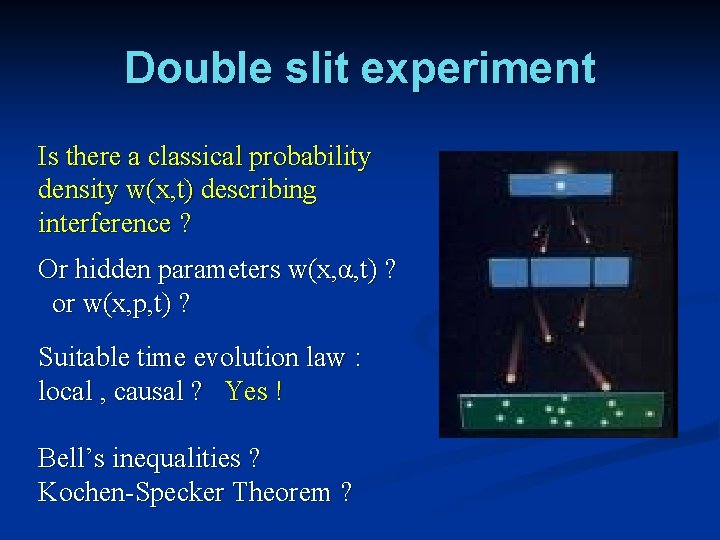Double slit experiment Is there a classical probability density w(x, t) describing interference ? Or hidden parameters w(x, α, t) ? or w(x, p, t) ? Suitable time evolution law : local , causal ? Yes ! Bell’s inequalities ? Kochen-Specker Theorem ?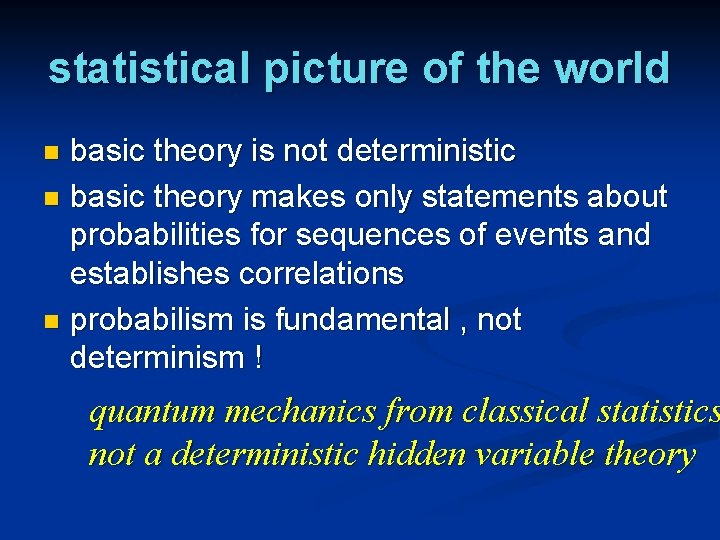statistical picture of the world basic theory is not deterministic n basic theory makes only statements about probabilities for sequences of events and establishes correlations n probabilism is fundamental , not determinism ! n quantum mechanics from classical statistics not a deterministic hidden variable theory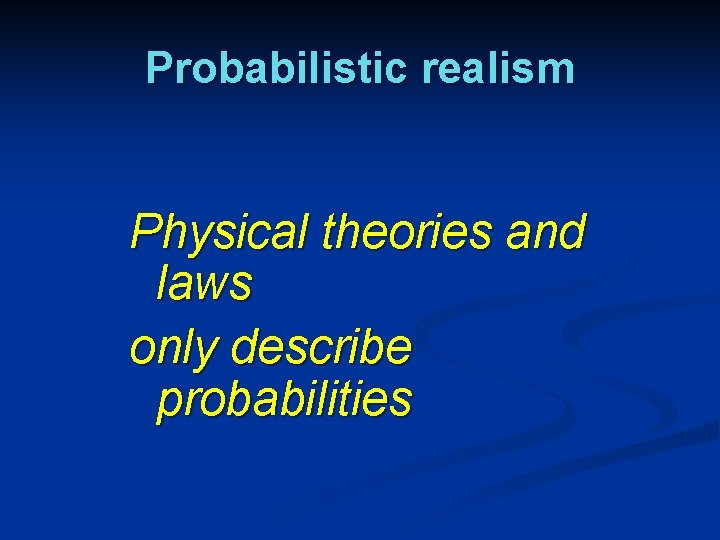Probabilistic realism Physical theories and laws only describe probabilitiesPhysics only describes probabilities Gott würfelt nicht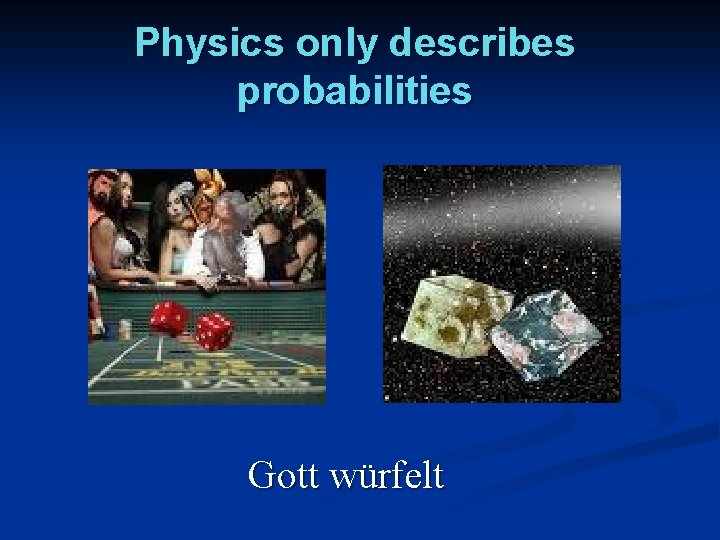Physics only describes probabilities Gott würfeltbut nothing beyond classical statistics is needed for quantum mechanics !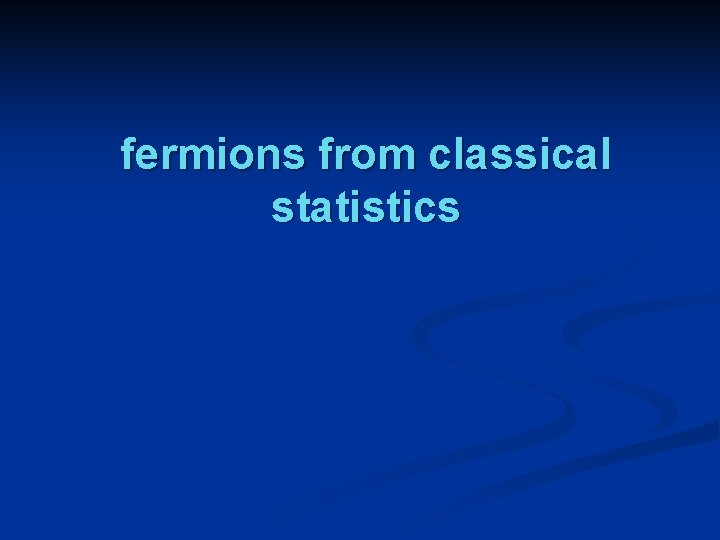fermions from classical statistics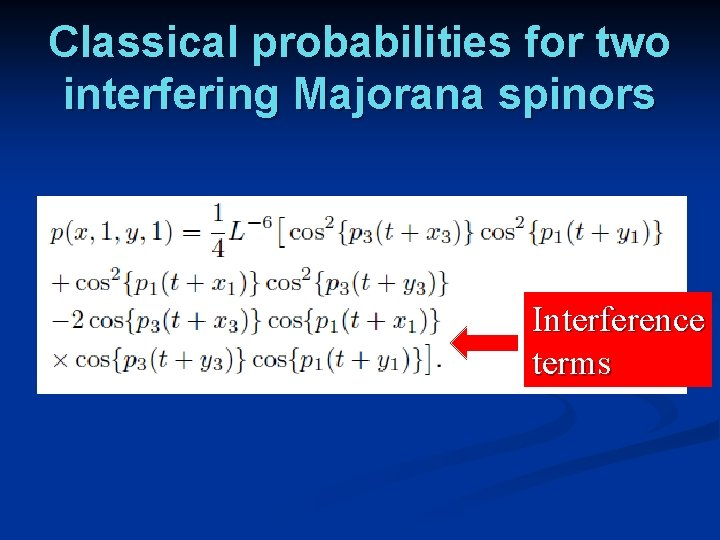Classical probabilities for two interfering Majorana spinors Interference terms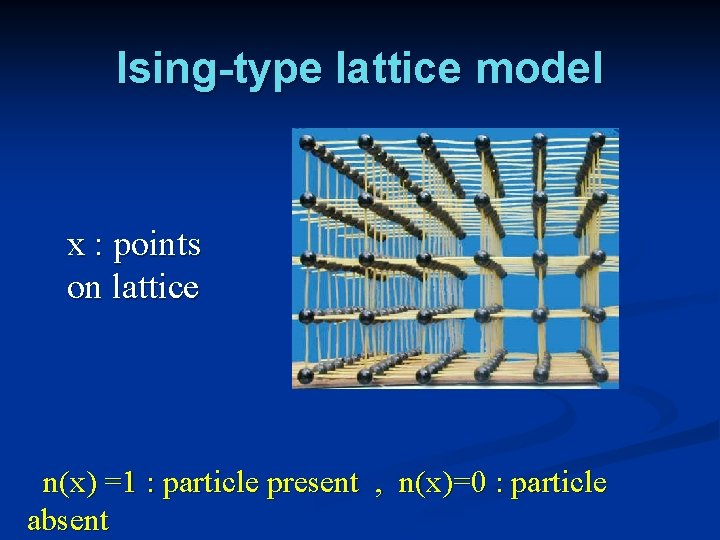Ising-type lattice model x : points on lattice n(x) =1 : particle present , n(x)=0 : particle absentmicrophysical ensemble n states τ n labeled by sequences of occupation numbers or bits ns = 0 or 1 n τ = [ ns ] = [0, 0, 1, 1, 1, 1, 0, …] etc. n s=(x, γ) n probabilities pτ > 0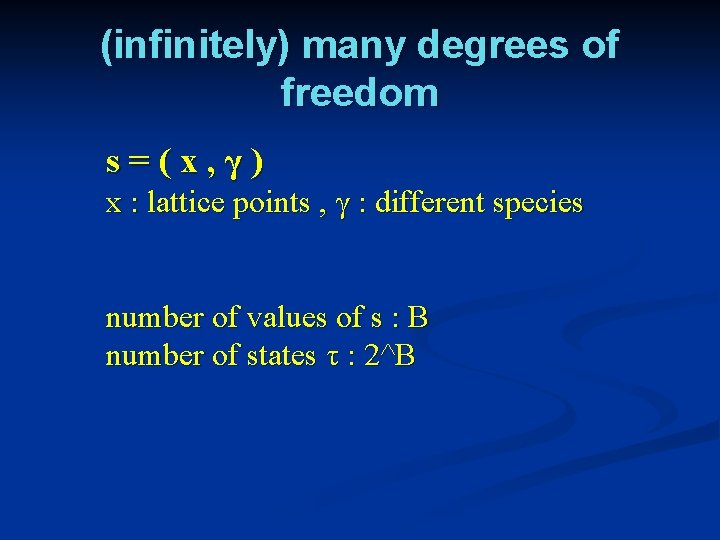(infinitely) many degrees of freedom s=(x, γ) x : lattice points , γ : different species number of values of s : B number of states τ : 2^BClassical wave function q is real , not necessarily positive Positivity of probabilities automatic.Time evolution Rotation preserves normalization of probabilities Evolution equation specifies dynamics simple evolution : R independent of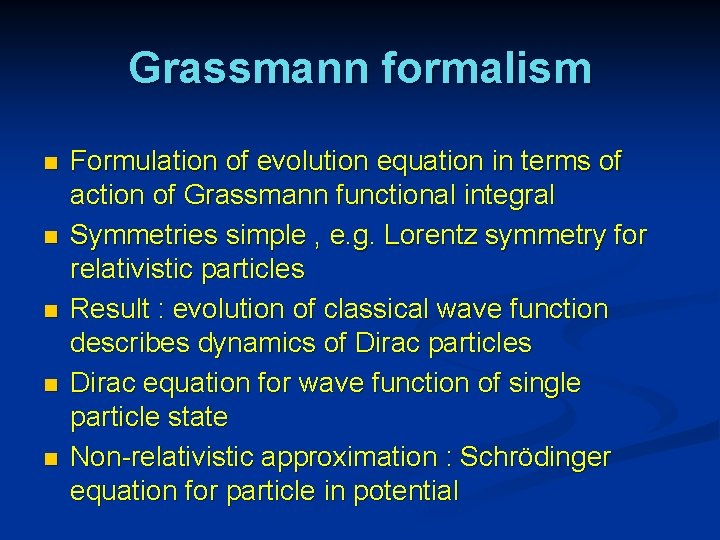Grassmann formalism n n n Formulation of evolution equation in terms of action of Grassmann functional integral Symmetries simple , e. g. Lorentz symmetry for relativistic particles Result : evolution of classical wave function describes dynamics of Dirac particles Dirac equation for wave function of single particle state Non-relativistic approximation : Schrödinger equation for particle in potentialGrassmann wave function Map between classical states and basis elements of Grassmann algebra s=(x, γ) For every ns= 0 : g τ contains factor ψs Grassmann wave function :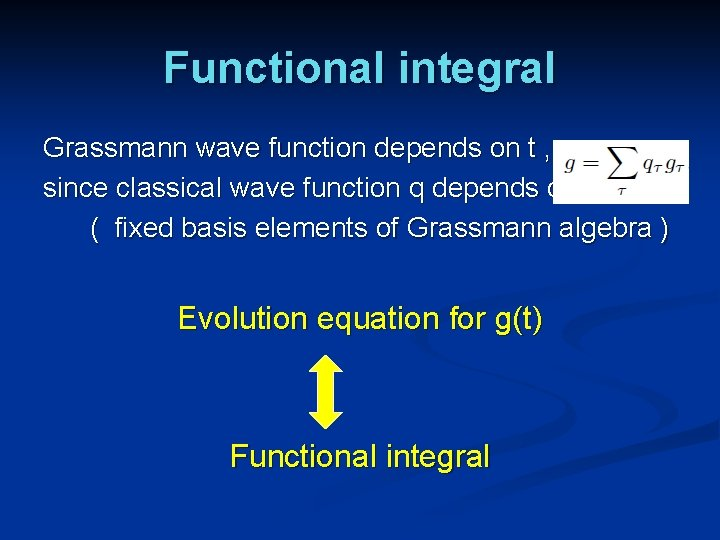Functional integral Grassmann wave function depends on t , since classical wave function q depends on t ( fixed basis elements of Grassmann algebra ) Evolution equation for g(t) Functional integralWave function from functional integral L(t) depends only on ψ(t) and ψ(t+ε)Evolution equation n Evolution equation for classical wave function , and therefore also for classical probability distribution , is specified by action S n Real Grassmann algebra needed , since classical wave function is realMassless Majorana spinors in four dimensionsTime evolution linear in q , non-linear in pOne particle states : arbitrary static “vacuum” state One –particle wave function obeys Dirac equation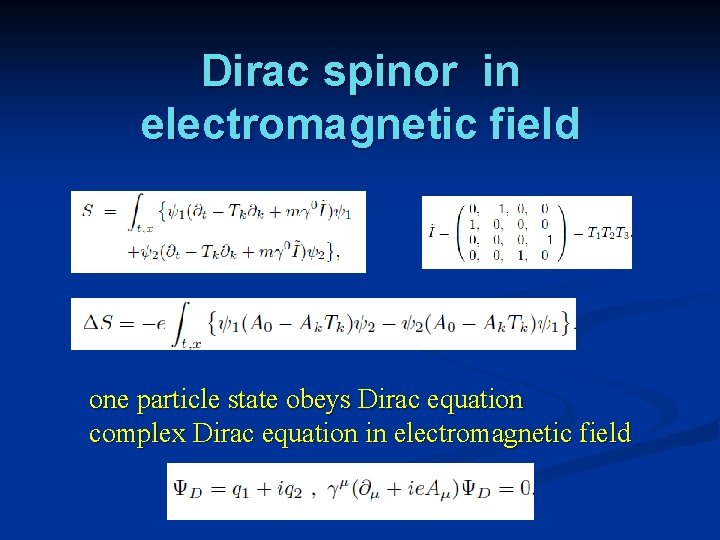Dirac spinor in electromagnetic field one particle state obeys Dirac equation complex Dirac equation in electromagnetic fieldSchrödinger equation Non – relativistic approximation : Time-evolution of particle in a potential described by standard Schrödinger equation. n Time evolution of probabilities in classical statistical Ising-type model generates all quantum features of particle in a potential , as interference ( double slit ) or tunneling. This holds if initial distribution corresponds to one-particle state. nquantum particle from classical probabilities ν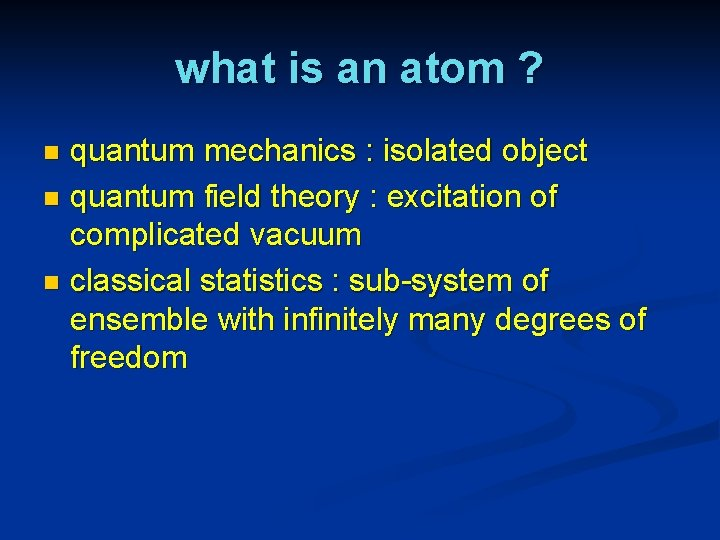what is an atom ? quantum mechanics : isolated object n quantum field theory : excitation of complicated vacuum n classical statistics : sub-system of ensemble with infinitely many degrees of freedom n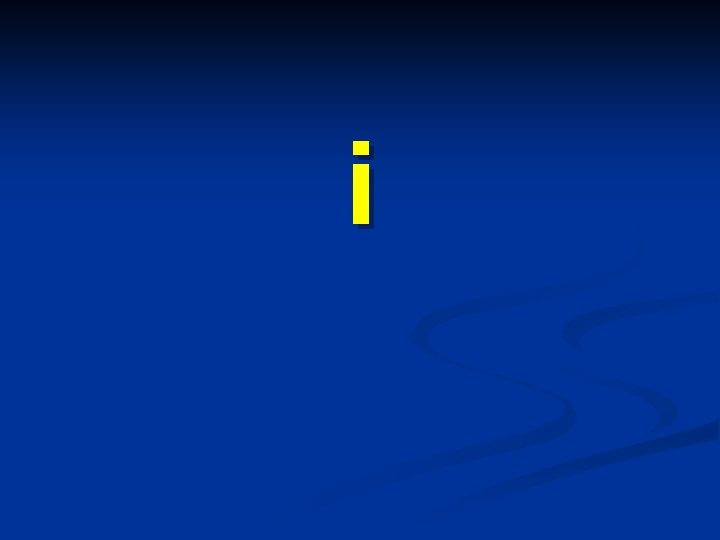iPhases and complex structure introduce complex spinors : complex wave function :hSimple conversion factor for unitsunitary time evolution νfermions and bosons ν[A, B]=Cnon-commuting observables classical statistical systems admit many product structures of observables n many different definitions of correlation functions possible , not only classical correlation ! n type of measurement determines correct selection of correlation function ! n example 1 : euclidean lattice gauge theories n example 2 : function observables n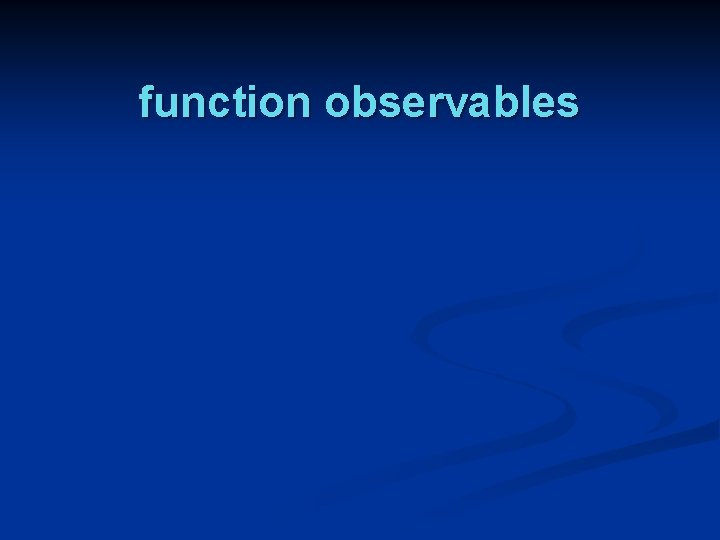function observables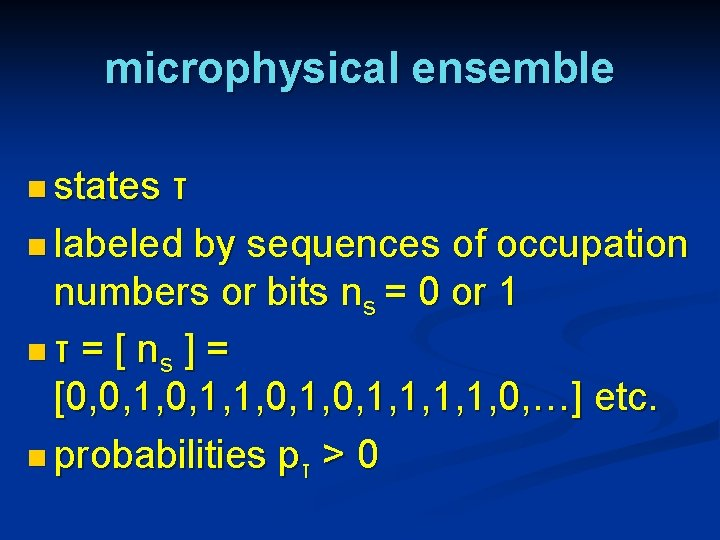microphysical ensemble n states τ n labeled by sequences of occupation numbers or bits ns = 0 or 1 n τ = [ ns ] = [0, 0, 1, 1, 1, 1, 0, …] etc. n probabilities pτ > 0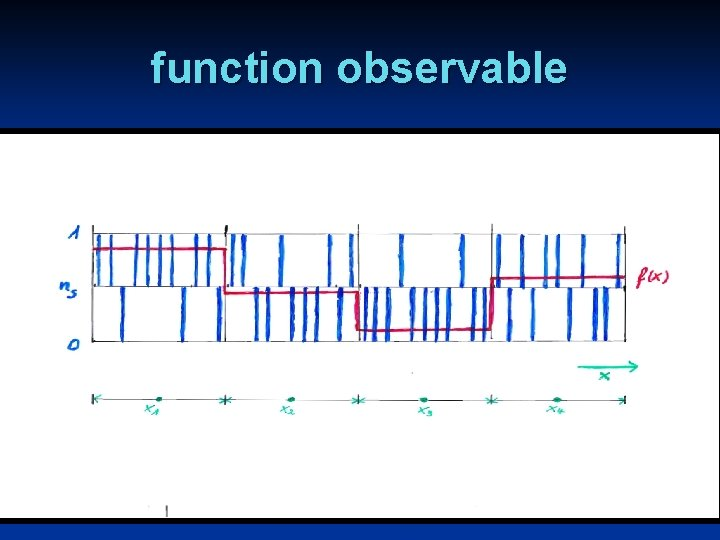function observable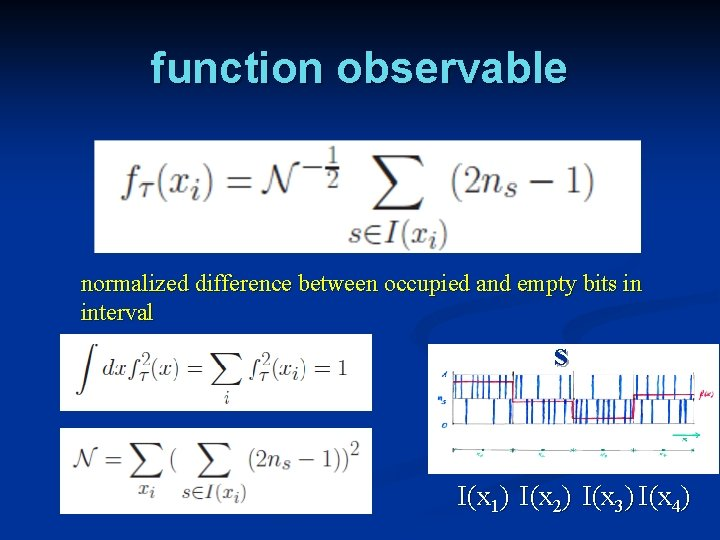function observable normalized difference between occupied and empty bits in interval s I(x 1) I(x 2) I(x 3) I(x 4)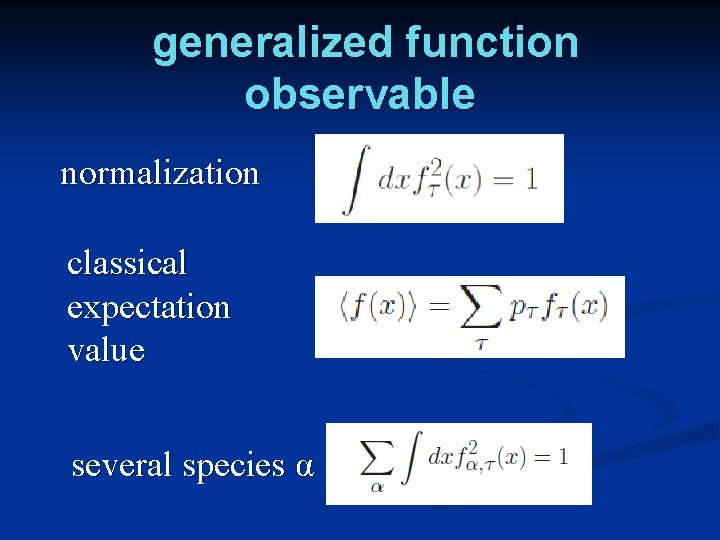generalized function observable normalization classical expectation value several species αposition classical observable : fixed value for every state τmomentum n derivative observable classical observable : fixed value for every state τcomplex structure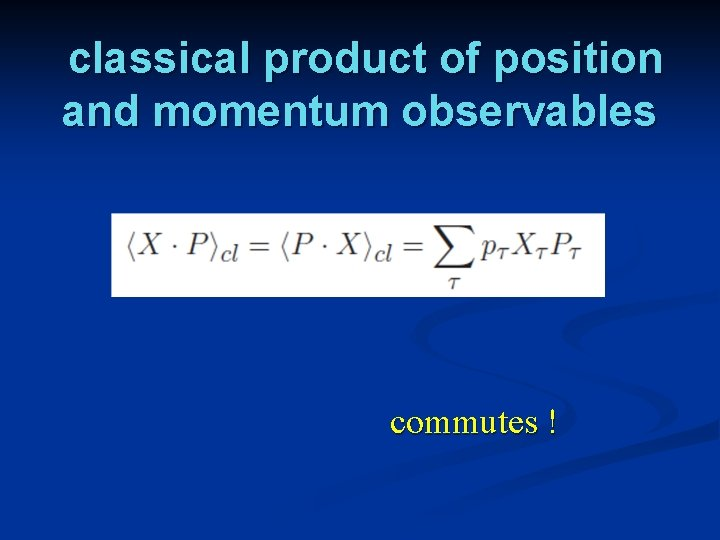classical product of position and momentum observables commutes !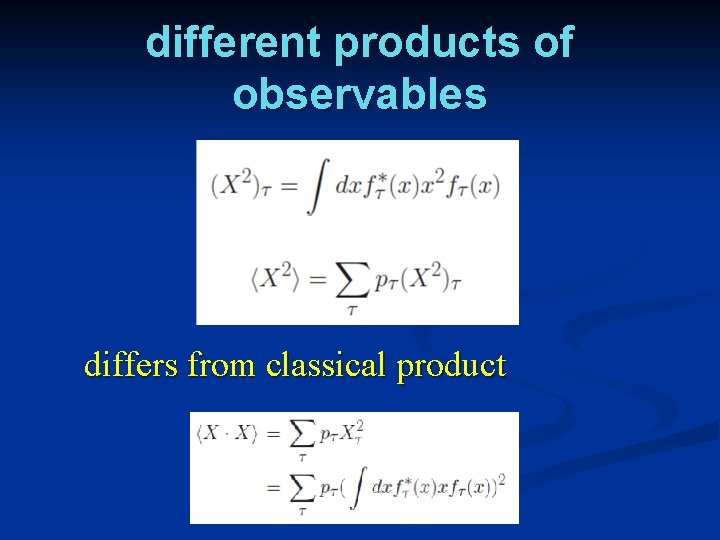different products of observables differs from classical product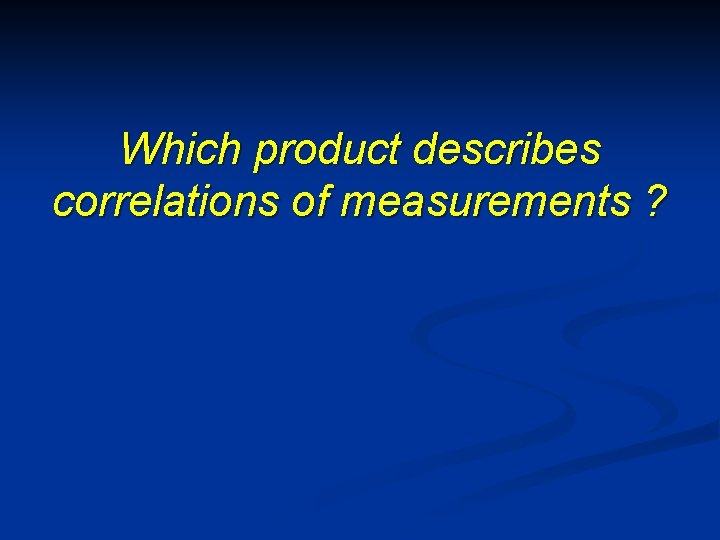Which product describes correlations of measurements ?coarse graining of information for subsystems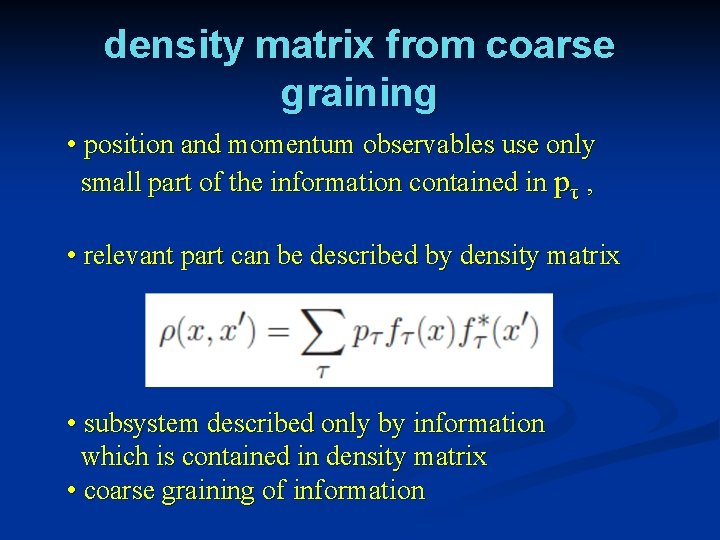density matrix from coarse graining • position and momentum observables use only small part of the information contained in pτ , • relevant part can be described by density matrix • subsystem described only by information which is contained in density matrix • coarse graining of informationquantum density matrix has the properties of a quantum density matrix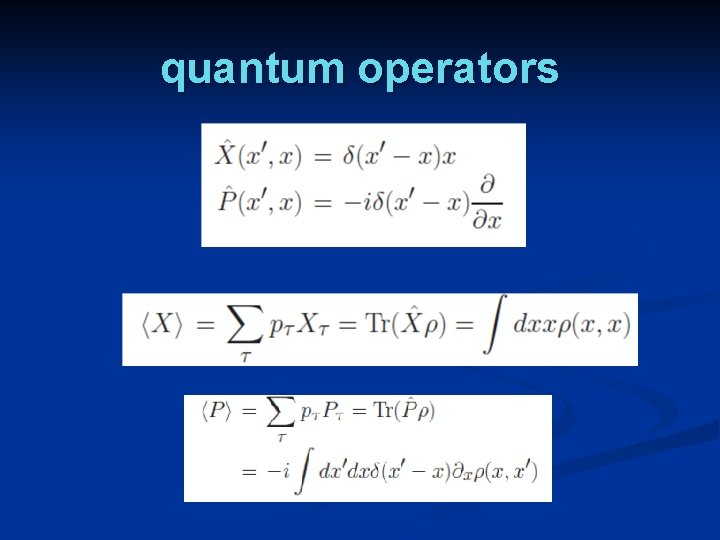quantum operators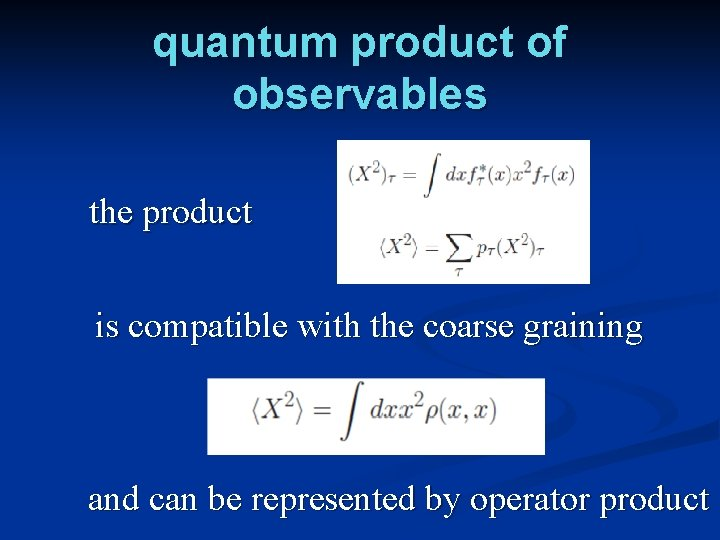quantum product of observables the product is compatible with the coarse graining and can be represented by operator product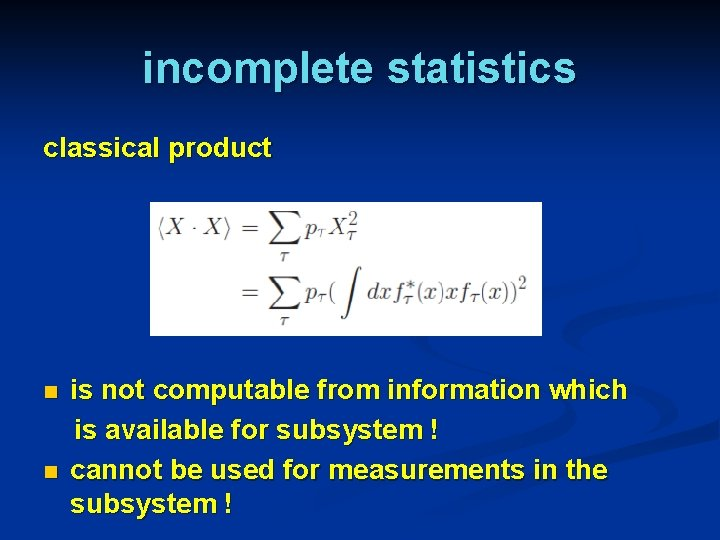incomplete statistics classical product n n is not computable from information which is available for subsystem ! cannot be used for measurements in the subsystem !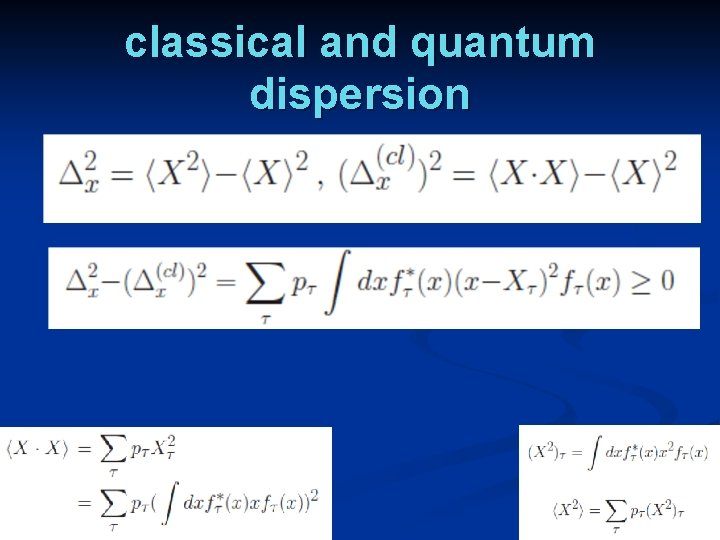classical and quantum dispersionsubsystem probabilities in contrast :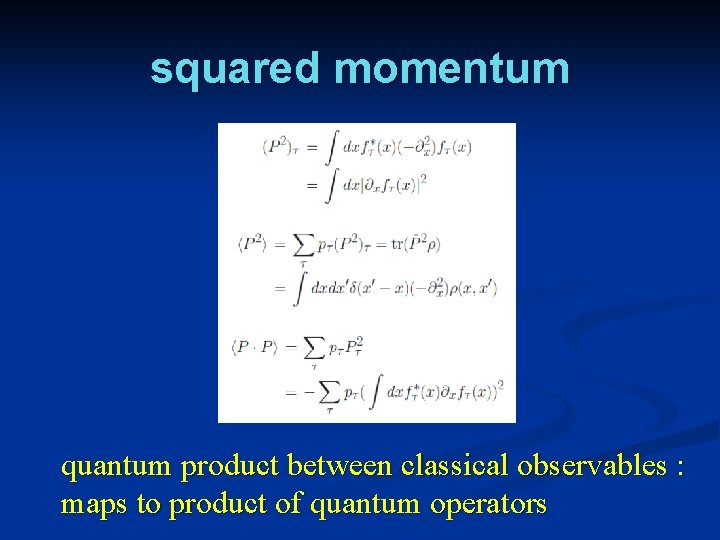squared momentum quantum product between classical observables : maps to product of quantum operatorsnon – commutativity in classical statistics commutator depends on choice of product !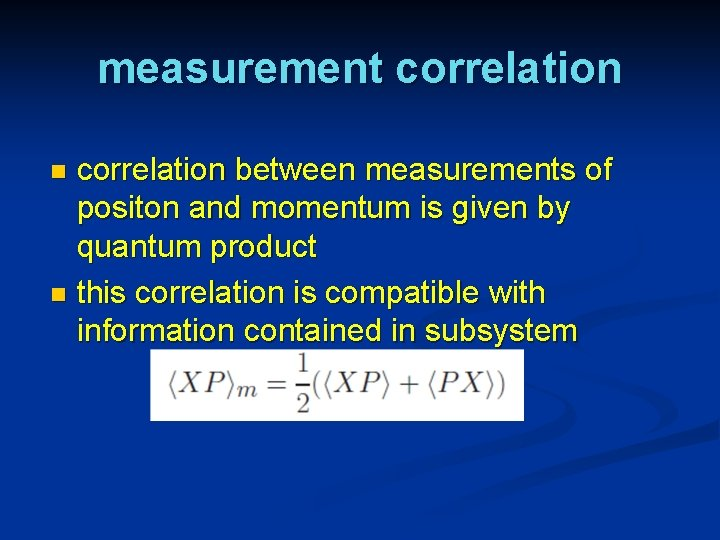measurement correlation between measurements of positon and momentum is given by quantum product n this correlation is compatible with information contained in subsystem n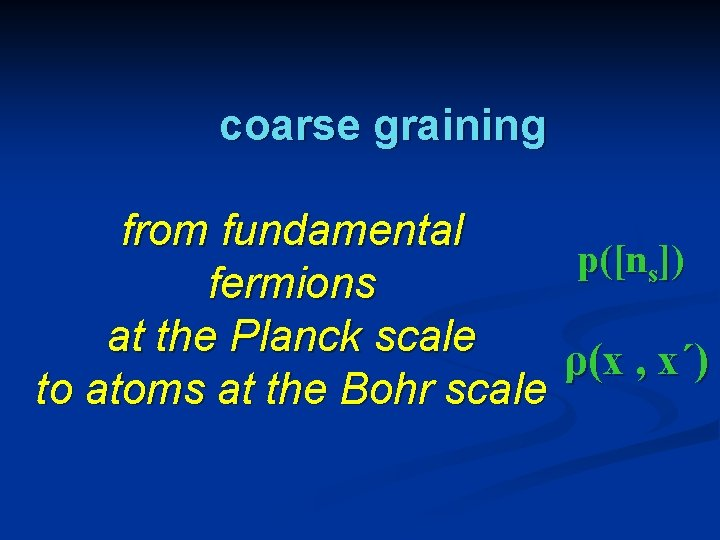coarse graining from fundamental p([ns]) fermions at the Planck scale ρ(x , x´) to atoms at the Bohr scale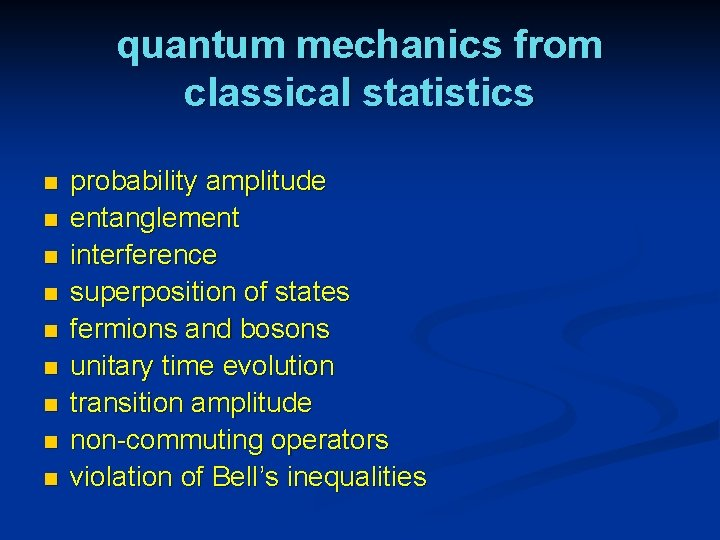quantum mechanics from classical statistics n n n n n probability amplitude entanglement interference superposition of states fermions and bosons unitary time evolution transition amplitude non-commuting operators violation of Bell’s inequalities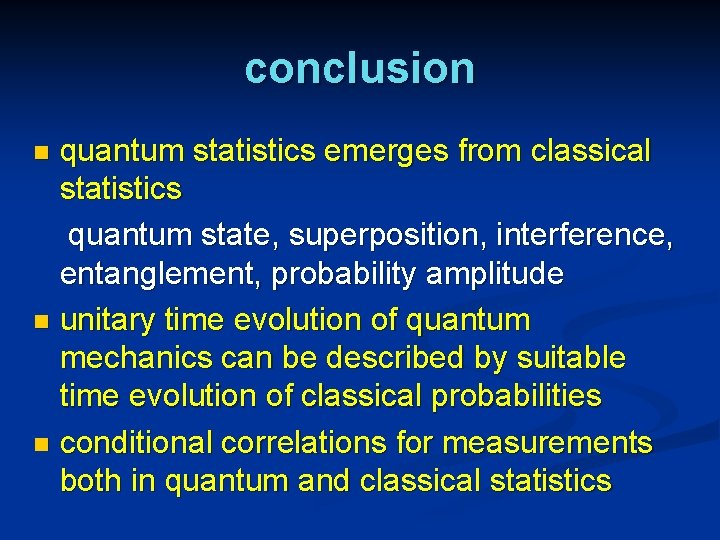conclusion quantum statistics emerges from classical statistics quantum state, superposition, interference, entanglement, probability amplitude n unitary time evolution of quantum mechanics can be described by suitable time evolution of classical probabilities n conditional correlations for measurements both in quantum and classical statistics nzwitters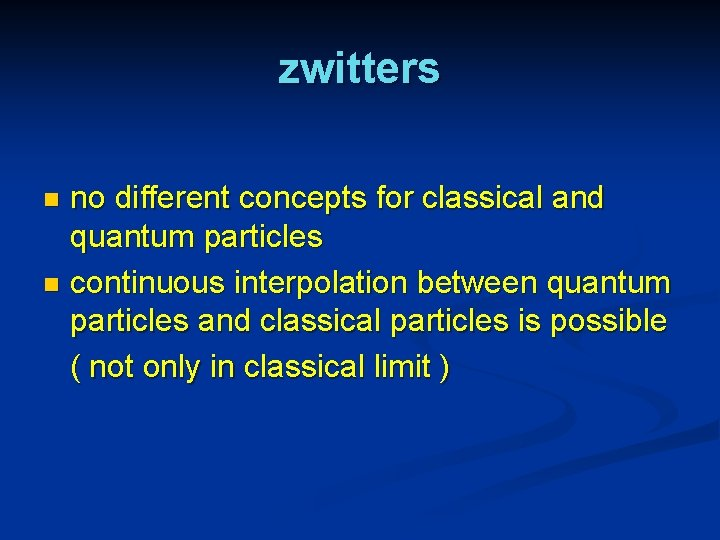zwitters no different concepts for classical and quantum particles n continuous interpolation between quantum particles and classical particles is possible ( not only in classical limit ) n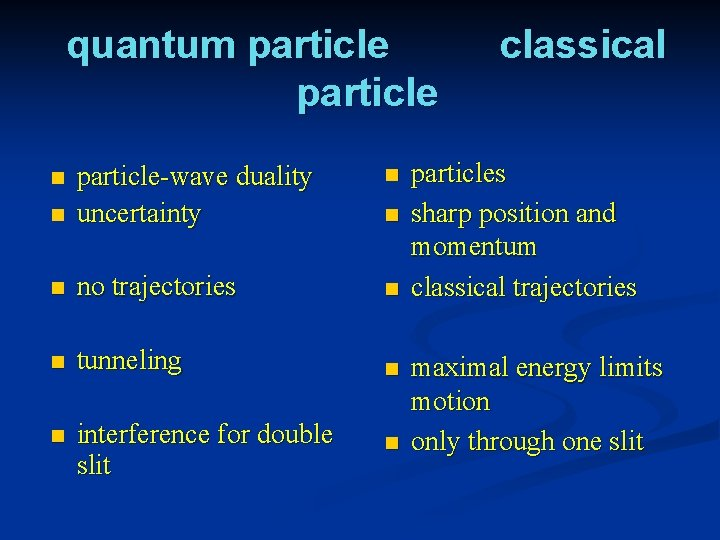quantum particle n n particle-wave duality uncertainty n no trajectories n n tunneling n n interference for double slit n n n classical particles sharp position and momentum classical trajectories maximal energy limits motion only through one slitquantum particle from classical probabilities in phase space n probability distribution in phase space for one particle w(x, p) n n as for classical particle ! observables different from classical observables time evolution of probability distribution different from the one for classical particle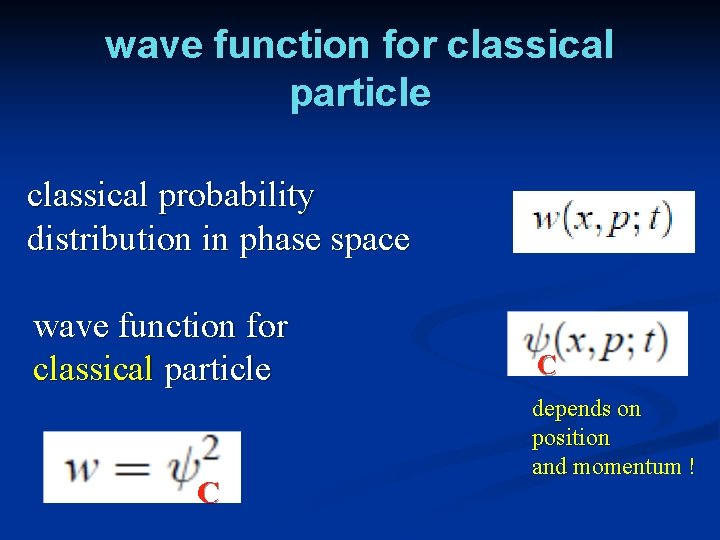wave function for classical particle classical probability distribution in phase space wave function for classical particle C C depends on position and momentum !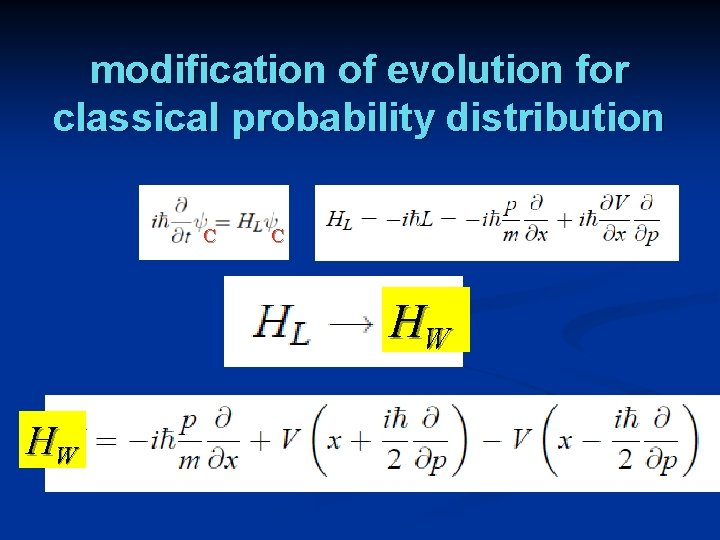modification of evolution for classical probability distribution C C HW HW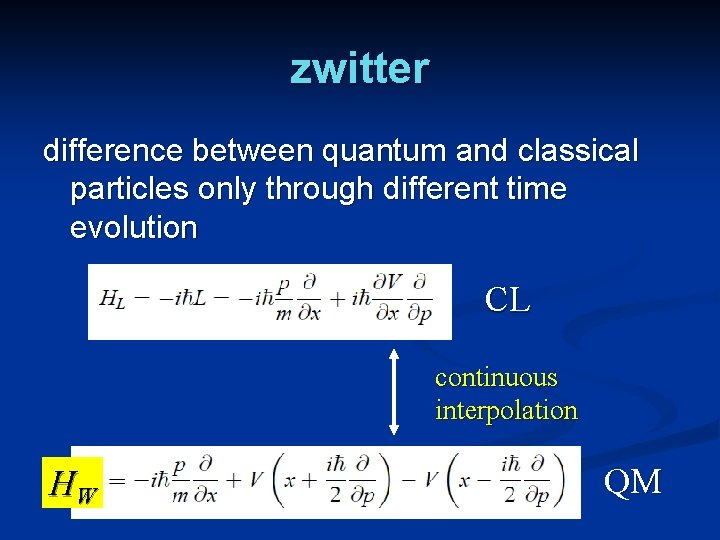zwitter difference between quantum and classical particles only through different time evolution CL continuous interpolation HW QM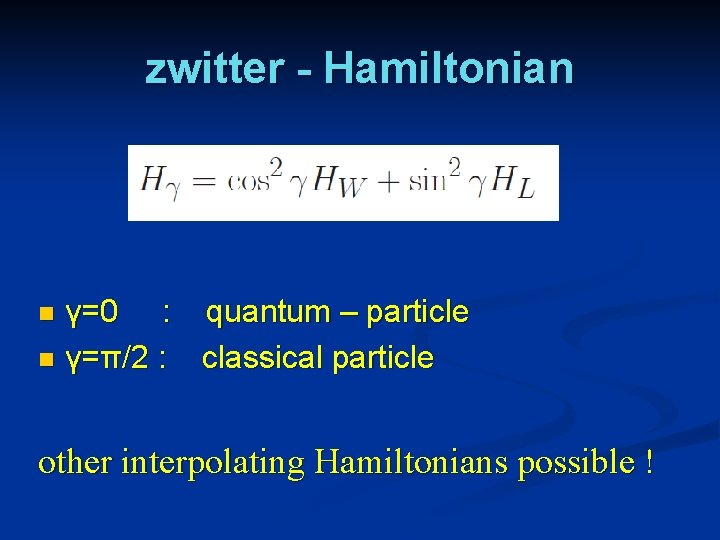zwitter - Hamiltonian γ=0 : quantum – particle n γ=π/2 : classical particle n other interpolating Hamiltonians possible !How good is quantum mechanics ? small parameter γ can be tested experimentally zwitter : no conserved microscopic energy C static state : orexperiments for determination or limits on zwitter – parameter γ ? lifetime of nuclear spin states > 60 h ( Heil et al. ) : γ < 10 -14Can quantum physics be described by classical probabilities ? “ No go “ theorems Bell , Clauser , Horne , Shimony , Holt implicit assumption : use of classical correlation function for correlation between measurements Kochen , Specker assumption : unique map from quantum operators to classical observables